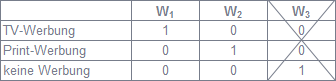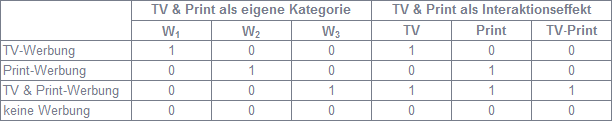# Categorical variables can be numbers

## Categorical variables in regression models

Johannes Lüken / Dr. Heiko Schimmelpfennig

Regression models are not limited to scale independent variables. Categorical variables such as gender, occupation, etc. can be taken into account if their characteristics are shown as numbers. Dummy coding is a common approach.

Dummy coding of independent dichotomous variables

The aim is to investigate what influence, in addition to the price, the placement of an advertisement has on monthly sales. The linear regression function is thus

Sales volume = b0 + b1× price + b2× advertising

While price is a metric variable, the advertisement has only two categories: an advertisement was placed (at the beginning of a month) or not. In order to take this influencing variable into account in the regression model, numbers must be assigned to both forms. If one follows the dummy coding, one reference category is given the value 0 and the other category the value 1. In this example, it makes sense to set the refrain from advertising as the reference category. The regression coefficient b2 then indicates exactly the amount by which the sales change due to the placement of an advertisement compared to the reference category “no advertisement” at a constant price.Dummy coding of independent variables with more than two categories

It is also differentiated whether a TV or print advertisement was placed. In this respect, three categories can be distinguished. The coding of the two variables W (erbung) therefore requires1 and advertising2 (see Figure 1).Figure 1: Dummy coding

The combination of the variables with the values ​​W1 = 1, W2 = 0 thus represents TV advertising, W1 = 0, W2 = 1 print advertisement and W1 = 0, W2 = 0 no advertising. These combinations clearly define all three categories. A third variable W3 would not only be redundant, but would lead to exact multicollinearity, so that the regression model would not be estimable. "No advertising" is the reference category here too, since both coding variables are 0 for these. In the corresponding regression function

Sales volume = b0 + b1× price + b2× W1 + b3× W2

quantify b2 and b3 the effects of TV or print advertising on sales volume compared to the reference category. The difference between b2 and b3 indicates how much the effect of an advertisement differs between the two media.

Effects of interaction with categorical variables

The interpretation of the regression coefficients assumes that there are no multiple answers for the categorical variable. This means that it must not have been advertised in TV and print in the same month. In order to determine the effect of joint advertising in both media, a separate additional category “TV & Print” must be taken into account (see Figure 2).

Alternatively, TV advertising (yes / no) and print advertising (yes / no) can be understood as two separate dichotomous variables. If one assumes that simultaneous advertising in both media does not have an additive effect, there is an interaction effect between them. This can be mapped in the model by including the product of the two variables:

Sales volume = b0 + b1× price + b2× TV + b3× Print + b4× TV × print

The effect of a joint advertisement is then equal to the sum of the individual effects and the interaction effect (b2 + b3 + b4). This corresponds to the regression coefficient of the coding variable W.3 from Figure 2, if TV & Print advertising is presented as a separate category.Figure 2: Dummy coding for multiple answers

An interaction effect between advertising and price, i.e. between a categorical and a metric variable, can also be taken into account in the model. In the introductory example of the dummy coding of the dichotomous variable advertising, your product with the price is included in the regression function:

Sales volume = b0 + b1× price + b2× advertising + b3× Price × Advertising

Assume that the relationship between price and sales volume is negative (b1 <0), a negative coefficient means b3that the effect of the price on sales is stronger when an advertisement is placed than without it. A positive coefficient, on the other hand, indicates lower price sensitivity.

In addition to dummy coding, effect coding and contrast coding are common procedures. The type of coding influences the regression coefficients and their interpretation. The coefficient of determination and thus the results of the tests of the significance of improvements in the coefficient of determination due to the consideration of further variables or of interaction effects are independent of this.

Contribution from planning & analysis 13/5 in the "Statistics compact" section

Author information

Johannes Lüken, Graduate psychologist, is head of the multivariate analysis department at IfaD, Institute for Applied Data Analysis, Hamburg. The focus of his work is the development of new methods, their implementation in analysis tools, as well as the application, training and advice with regard to these processes.

Prof. Dr. Heiko Schimmelpfennig is project manager for multivariate analyzes at IfaD, Institute for Applied Data Analysis, and Professor of Business Administration at BiTS, Business and Information Technology School, Hamburg. At IfaD, he is primarily responsible for the advice, application and training of these procedures and represents the field of quantitative methods in economics in teaching.

literature

Cohen, J .; Cohen, P .; West, S. G .; Aiken, L. S .: Interactions With Categorical Variables, In: Applied Multiple Regression / Correlation Analysis for the Behavioral Sciences, 3rd Edition, Mahwah, New Jersey, 2003, pp. 354-389.

Eid, M .; Gollwitzer, M .; Schmitt, M ​​.: Multiple Regression Analysis, In: Statistics and Research Methods, 2nd Edition, Weinheim, Basel, 2011, pp. 648-677.

### The department